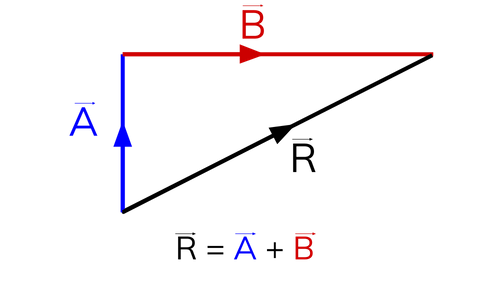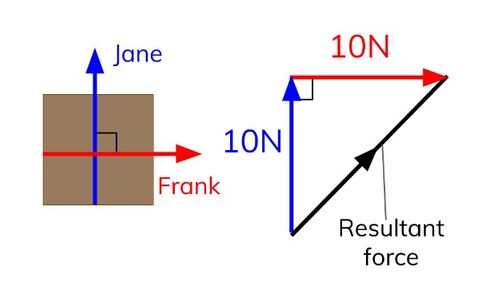# The Basics of Motion - Physics Paper 2

?

Speed

• Speed is a scalar quantity.

• Scalar quantities only have a magnitude (size).

• Scalar quantities, like speed, do not have a direction.

Vector

• Velocity describes an object’s direction as well as its speed.

• Velocity is a vector quantity because it has a magnitude (or size) and a direction.

Typical Speeds

• Typical walking speed is 1.4m/s.

• Typical running speed is 3.3m/s.

• Typical speed of a bus in town is 10m/s.

• Typical speed of a car on the motorway is 31m/s.

• Typical speed of wind is 6m/s.

• Typical speed of sound is 340m/s.• To add vectors, draw each vector as an arrow one after the other.

• The length of the arrow represents the magnitude (size) of a quantity.

• The direction of the arrow represents the direction of the vector quantity.• Jane pushes a table with a force of 10N towards the door, but her brother, Frank tries to stop her by pushing it with a force of 10 N at a right angle to Jane.

• The effect of these forces is shown above.

• Pythagoras’ theorem is used to work out the resultant force.

• Resultant Force = √(10